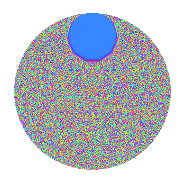# Properties

 Label 2.74.a.aLevel 2 Weight 74 Character orbit 2.a Self dual Yes Analytic conductor 67.497 Analytic rank 1 Dimension 3 CM No Inner twists 1

# Related objects

## Newspace parameters

 Level: $$N$$ = $$2$$ Weight: $$k$$ = $$74$$ Character orbit: $$[\chi]$$ = 2.a (trivial)

## Newform invariants

 Self dual: Yes Analytic conductor: $$67.4967947474$$ Analytic rank: $$1$$ Dimension: $$3$$ Coefficient field: $$\mathbb{Q}[x]/(x^{3} - \cdots)$$ Coefficient ring: $$\Z[a_1, \ldots, a_{7}]$$ Coefficient ring index: $$2^{23}\cdot 3^{7}\cdot 5^{3}$$ Fricke sign: $$1$$ Sato-Tate group: $\mathrm{SU}(2)$

## $q$-expansion

Coefficients of the $$q$$-expansion are expressed in terms of a basis $$1,\beta_1,\beta_2$$ for the coefficient ring described below. We also show the integral $$q$$-expansion of the trace form.

 $$f(q)$$ $$=$$ $$q -68719476736 q^{2} +(-101346400339911324 + \beta_{1}) q^{3} +$$$$47\!\cdots\!96$$$$q^{4} +(-$$$$10\!\cdots\!50$$$$+ 16642465 \beta_{1} - 7 \beta_{2}) q^{5} +($$$$69\!\cdots\!64$$$$- 68719476736 \beta_{1}) q^{6} +(-$$$$77\!\cdots\!88$$$$+ 7903338308822 \beta_{1} - 1662860 \beta_{2}) q^{7} -$$$$32\!\cdots\!56$$$$q^{8} +($$$$24\!\cdots\!53$$$$- 16762740084667458 \beta_{1} + 4620715470 \beta_{2}) q^{9} +O(q^{10})$$ $$q -68719476736 q^{2} +(-101346400339911324 + \beta_{1}) q^{3} +$$$$47\!\cdots\!96$$$$q^{4} +(-$$$$10\!\cdots\!50$$$$+ 16642465 \beta_{1} - 7 \beta_{2}) q^{5} +($$$$69\!\cdots\!64$$$$- 68719476736 \beta_{1}) q^{6} +(-$$$$77\!\cdots\!88$$$$+ 7903338308822 \beta_{1} - 1662860 \beta_{2}) q^{7} -$$$$32\!\cdots\!56$$$$q^{8} +($$$$24\!\cdots\!53$$$$- 16762740084667458 \beta_{1} + 4620715470 \beta_{2}) q^{9} +($$$$74\!\cdots\!00$$$$- 1143661486397194240 \beta_{1} + 481036337152 \beta_{2}) q^{10} +(-$$$$26\!\cdots\!48$$$$+ 38059855453876711147 \beta_{1} + 15290845419080 \beta_{2}) q^{11} +(-$$$$47\!\cdots\!04$$$$+$$$$47\!\cdots\!96$$$$\beta_{1}) q^{12} +($$$$50\!\cdots\!46$$$$+$$$$87\!\cdots\!57$$$$\beta_{1} - 2079791218114015 \beta_{2}) q^{13} +($$$$53\!\cdots\!68$$$$-$$$$54\!\cdots\!92$$$$\beta_{1} + 114270869085224960 \beta_{2}) q^{14} +($$$$24\!\cdots\!00$$$$-$$$$19\!\cdots\!10$$$$\beta_{1} + 2087835322029899148 \beta_{2}) q^{15} +$$$$22\!\cdots\!16$$$$q^{16} +(-$$$$67\!\cdots\!78$$$$+$$$$12\!\cdots\!38$$$$\beta_{1} - 69779524841156108930 \beta_{2}) q^{17} +(-$$$$17\!\cdots\!08$$$$+$$$$11\!\cdots\!88$$$$\beta_{1} -$$$$31\!\cdots\!20$$$$\beta_{2}) q^{18} +(-$$$$13\!\cdots\!60$$$$-$$$$80\!\cdots\!03$$$$\beta_{1} +$$$$32\!\cdots\!20$$$$\beta_{2}) q^{19} +(-$$$$51\!\cdots\!00$$$$+$$$$78\!\cdots\!40$$$$\beta_{1} -$$$$33\!\cdots\!72$$$$\beta_{2}) q^{20} +($$$$72\!\cdots\!12$$$$-$$$$24\!\cdots\!36$$$$\beta_{1} +$$$$51\!\cdots\!80$$$$\beta_{2}) q^{21} +($$$$18\!\cdots\!28$$$$-$$$$26\!\cdots\!92$$$$\beta_{1} -$$$$10\!\cdots\!80$$$$\beta_{2}) q^{22} +($$$$26\!\cdots\!96$$$$-$$$$19\!\cdots\!94$$$$\beta_{1} -$$$$62\!\cdots\!60$$$$\beta_{2}) q^{23} +($$$$32\!\cdots\!44$$$$-$$$$32\!\cdots\!56$$$$\beta_{1}) q^{24} +($$$$32\!\cdots\!75$$$$-$$$$34\!\cdots\!00$$$$\beta_{1} +$$$$12\!\cdots\!00$$$$\beta_{2}) q^{25} +(-$$$$34\!\cdots\!56$$$$-$$$$60\!\cdots\!52$$$$\beta_{1} +$$$$14\!\cdots\!40$$$$\beta_{2}) q^{26} +($$$$29\!\cdots\!80$$$$-$$$$37\!\cdots\!98$$$$\beta_{1} -$$$$14\!\cdots\!40$$$$\beta_{2}) q^{27} +(-$$$$36\!\cdots\!48$$$$+$$$$37\!\cdots\!12$$$$\beta_{1} -$$$$78\!\cdots\!60$$$$\beta_{2}) q^{28} +($$$$55\!\cdots\!70$$$$+$$$$64\!\cdots\!49$$$$\beta_{1} +$$$$53\!\cdots\!45$$$$\beta_{2}) q^{29} +(-$$$$17\!\cdots\!00$$$$+$$$$13\!\cdots\!60$$$$\beta_{1} -$$$$14\!\cdots\!28$$$$\beta_{2}) q^{30} +($$$$31\!\cdots\!32$$$$+$$$$27\!\cdots\!04$$$$\beta_{1} +$$$$35\!\cdots\!80$$$$\beta_{2}) q^{31} -$$$$15\!\cdots\!76$$$$q^{32} +($$$$58\!\cdots\!52$$$$-$$$$17\!\cdots\!46$$$$\beta_{1} -$$$$42\!\cdots\!30$$$$\beta_{2}) q^{33} +($$$$46\!\cdots\!08$$$$-$$$$86\!\cdots\!68$$$$\beta_{1} +$$$$47\!\cdots\!80$$$$\beta_{2}) q^{34} +($$$$31\!\cdots\!00$$$$-$$$$86\!\cdots\!20$$$$\beta_{1} +$$$$22\!\cdots\!76$$$$\beta_{2}) q^{35} +($$$$11\!\cdots\!88$$$$-$$$$79\!\cdots\!68$$$$\beta_{1} +$$$$21\!\cdots\!20$$$$\beta_{2}) q^{36} +($$$$31\!\cdots\!22$$$$+$$$$47\!\cdots\!73$$$$\beta_{1} -$$$$28\!\cdots\!55$$$$\beta_{2}) q^{37} +($$$$95\!\cdots\!60$$$$+$$$$55\!\cdots\!08$$$$\beta_{1} -$$$$22\!\cdots\!20$$$$\beta_{2}) q^{38} +($$$$66\!\cdots\!96$$$$+$$$$94\!\cdots\!58$$$$\beta_{1} +$$$$10\!\cdots\!00$$$$\beta_{2}) q^{39} +($$$$35\!\cdots\!00$$$$-$$$$54\!\cdots\!40$$$$\beta_{1} +$$$$22\!\cdots\!92$$$$\beta_{2}) q^{40} +($$$$50\!\cdots\!82$$$$-$$$$72\!\cdots\!24$$$$\beta_{1} -$$$$17\!\cdots\!40$$$$\beta_{2}) q^{41} +(-$$$$50\!\cdots\!32$$$$+$$$$17\!\cdots\!96$$$$\beta_{1} -$$$$35\!\cdots\!80$$$$\beta_{2}) q^{42} +(-$$$$15\!\cdots\!64$$$$-$$$$16\!\cdots\!17$$$$\beta_{1} +$$$$55\!\cdots\!40$$$$\beta_{2}) q^{43} +(-$$$$12\!\cdots\!08$$$$+$$$$17\!\cdots\!12$$$$\beta_{1} +$$$$72\!\cdots\!80$$$$\beta_{2}) q^{44} +(-$$$$11\!\cdots\!50$$$$+$$$$26\!\cdots\!45$$$$\beta_{1} -$$$$21\!\cdots\!11$$$$\beta_{2}) q^{45} +(-$$$$18\!\cdots\!56$$$$+$$$$13\!\cdots\!84$$$$\beta_{1} +$$$$42\!\cdots\!60$$$$\beta_{2}) q^{46} +(-$$$$41\!\cdots\!88$$$$-$$$$34\!\cdots\!68$$$$\beta_{1} -$$$$10\!\cdots\!20$$$$\beta_{2}) q^{47} +(-$$$$22\!\cdots\!84$$$$+$$$$22\!\cdots\!16$$$$\beta_{1}) q^{48} +($$$$26\!\cdots\!37$$$$-$$$$19\!\cdots\!12$$$$\beta_{1} +$$$$38\!\cdots\!40$$$$\beta_{2}) q^{49} +(-$$$$22\!\cdots\!00$$$$+$$$$23\!\cdots\!00$$$$\beta_{1} -$$$$87\!\cdots\!00$$$$\beta_{2}) q^{50} +($$$$17\!\cdots\!72$$$$-$$$$66\!\cdots\!70$$$$\beta_{1} +$$$$25\!\cdots\!80$$$$\beta_{2}) q^{51} +($$$$23\!\cdots\!16$$$$+$$$$41\!\cdots\!72$$$$\beta_{1} -$$$$98\!\cdots\!40$$$$\beta_{2}) q^{52} +($$$$47\!\cdots\!86$$$$+$$$$41\!\cdots\!17$$$$\beta_{1} -$$$$12\!\cdots\!55$$$$\beta_{2}) q^{53} +(-$$$$20\!\cdots\!80$$$$+$$$$25\!\cdots\!28$$$$\beta_{1} +$$$$96\!\cdots\!40$$$$\beta_{2}) q^{54} +(-$$$$23\!\cdots\!00$$$$+$$$$53\!\cdots\!30$$$$\beta_{1} +$$$$17\!\cdots\!96$$$$\beta_{2}) q^{55} +($$$$25\!\cdots\!28$$$$-$$$$25\!\cdots\!32$$$$\beta_{1} +$$$$53\!\cdots\!60$$$$\beta_{2}) q^{56} +(-$$$$52\!\cdots\!60$$$$-$$$$20\!\cdots\!58$$$$\beta_{1} -$$$$46\!\cdots\!90$$$$\beta_{2}) q^{57} +(-$$$$38\!\cdots\!20$$$$-$$$$44\!\cdots\!64$$$$\beta_{1} -$$$$36\!\cdots\!20$$$$\beta_{2}) q^{58} +(-$$$$12\!\cdots\!60$$$$+$$$$26\!\cdots\!83$$$$\beta_{1} +$$$$31\!\cdots\!40$$$$\beta_{2}) q^{59} +($$$$11\!\cdots\!00$$$$-$$$$91\!\cdots\!60$$$$\beta_{1} +$$$$98\!\cdots\!08$$$$\beta_{2}) q^{60} +(-$$$$44\!\cdots\!38$$$$+$$$$45\!\cdots\!73$$$$\beta_{1} +$$$$13\!\cdots\!85$$$$\beta_{2}) q^{61} +(-$$$$21\!\cdots\!52$$$$-$$$$18\!\cdots\!44$$$$\beta_{1} -$$$$24\!\cdots\!80$$$$\beta_{2}) q^{62} +(-$$$$22\!\cdots\!64$$$$+$$$$71\!\cdots\!30$$$$\beta_{1} -$$$$46\!\cdots\!60$$$$\beta_{2}) q^{63} +$$$$10\!\cdots\!36$$$$q^{64} +($$$$43\!\cdots\!00$$$$-$$$$23\!\cdots\!60$$$$\beta_{1} +$$$$93\!\cdots\!88$$$$\beta_{2}) q^{65} +(-$$$$40\!\cdots\!72$$$$+$$$$12\!\cdots\!56$$$$\beta_{1} +$$$$28\!\cdots\!80$$$$\beta_{2}) q^{66} +(-$$$$33\!\cdots\!48$$$$-$$$$10\!\cdots\!59$$$$\beta_{1} -$$$$45\!\cdots\!20$$$$\beta_{2}) q^{67} +(-$$$$31\!\cdots\!88$$$$+$$$$59\!\cdots\!48$$$$\beta_{1} -$$$$32\!\cdots\!80$$$$\beta_{2}) q^{68} +(-$$$$19\!\cdots\!04$$$$+$$$$55\!\cdots\!92$$$$\beta_{1} +$$$$86\!\cdots\!60$$$$\beta_{2}) q^{69} +(-$$$$21\!\cdots\!00$$$$+$$$$59\!\cdots\!20$$$$\beta_{1} -$$$$15\!\cdots\!36$$$$\beta_{2}) q^{70} +(-$$$$38\!\cdots\!08$$$$+$$$$33\!\cdots\!58$$$$\beta_{1} +$$$$19\!\cdots\!80$$$$\beta_{2}) q^{71} +(-$$$$81\!\cdots\!68$$$$+$$$$54\!\cdots\!48$$$$\beta_{1} -$$$$14\!\cdots\!20$$$$\beta_{2}) q^{72} +(-$$$$51\!\cdots\!94$$$$-$$$$20\!\cdots\!98$$$$\beta_{1} +$$$$21\!\cdots\!70$$$$\beta_{2}) q^{73} +(-$$$$21\!\cdots\!92$$$$-$$$$32\!\cdots\!28$$$$\beta_{1} +$$$$19\!\cdots\!80$$$$\beta_{2}) q^{74} +(-$$$$31\!\cdots\!00$$$$+$$$$21\!\cdots\!75$$$$\beta_{1} -$$$$52\!\cdots\!00$$$$\beta_{2}) q^{75} +(-$$$$65\!\cdots\!60$$$$-$$$$37\!\cdots\!88$$$$\beta_{1} +$$$$15\!\cdots\!20$$$$\beta_{2}) q^{76} +(-$$$$60\!\cdots\!76$$$$+$$$$13\!\cdots\!68$$$$\beta_{1} +$$$$59\!\cdots\!20$$$$\beta_{2}) q^{77} +(-$$$$45\!\cdots\!56$$$$-$$$$64\!\cdots\!88$$$$\beta_{1} -$$$$68\!\cdots\!00$$$$\beta_{2}) q^{78} +(-$$$$13\!\cdots\!00$$$$+$$$$26\!\cdots\!92$$$$\beta_{1} +$$$$22\!\cdots\!40$$$$\beta_{2}) q^{79} +(-$$$$24\!\cdots\!00$$$$+$$$$37\!\cdots\!40$$$$\beta_{1} -$$$$15\!\cdots\!12$$$$\beta_{2}) q^{80} +(-$$$$50\!\cdots\!39$$$$-$$$$10\!\cdots\!54$$$$\beta_{1} -$$$$81\!\cdots\!10$$$$\beta_{2}) q^{81} +(-$$$$34\!\cdots\!52$$$$+$$$$49\!\cdots\!64$$$$\beta_{1} +$$$$11\!\cdots\!40$$$$\beta_{2}) q^{82} +($$$$16\!\cdots\!96$$$$-$$$$25\!\cdots\!55$$$$\beta_{1} -$$$$23\!\cdots\!80$$$$\beta_{2}) q^{83} +($$$$34\!\cdots\!52$$$$-$$$$11\!\cdots\!56$$$$\beta_{1} +$$$$24\!\cdots\!80$$$$\beta_{2}) q^{84} +($$$$21\!\cdots\!00$$$$-$$$$63\!\cdots\!70$$$$\beta_{1} +$$$$67\!\cdots\!86$$$$\beta_{2}) q^{85} +($$$$10\!\cdots\!04$$$$+$$$$11\!\cdots\!12$$$$\beta_{1} -$$$$38\!\cdots\!40$$$$\beta_{2}) q^{86} +($$$$47\!\cdots\!20$$$$+$$$$18\!\cdots\!54$$$$\beta_{1} -$$$$12\!\cdots\!00$$$$\beta_{2}) q^{87} +($$$$86\!\cdots\!88$$$$-$$$$12\!\cdots\!32$$$$\beta_{1} -$$$$49\!\cdots\!80$$$$\beta_{2}) q^{88} +($$$$15\!\cdots\!90$$$$-$$$$48\!\cdots\!90$$$$\beta_{1} +$$$$15\!\cdots\!50$$$$\beta_{2}) q^{89} +($$$$76\!\cdots\!00$$$$-$$$$18\!\cdots\!20$$$$\beta_{1} +$$$$14\!\cdots\!96$$$$\beta_{2}) q^{90} +($$$$14\!\cdots\!52$$$$-$$$$32\!\cdots\!44$$$$\beta_{1} +$$$$21\!\cdots\!40$$$$\beta_{2}) q^{91} +($$$$12\!\cdots\!16$$$$-$$$$94\!\cdots\!24$$$$\beta_{1} -$$$$29\!\cdots\!60$$$$\beta_{2}) q^{92} +($$$$19\!\cdots\!32$$$$+$$$$10\!\cdots\!96$$$$\beta_{1} -$$$$89\!\cdots\!40$$$$\beta_{2}) q^{93} +($$$$28\!\cdots\!68$$$$+$$$$23\!\cdots\!48$$$$\beta_{1} +$$$$72\!\cdots\!20$$$$\beta_{2}) q^{94} +(-$$$$17\!\cdots\!00$$$$+$$$$13\!\cdots\!50$$$$\beta_{1} -$$$$15\!\cdots\!20$$$$\beta_{2}) q^{95} +($$$$15\!\cdots\!24$$$$-$$$$15\!\cdots\!76$$$$\beta_{1}) q^{96} +($$$$25\!\cdots\!42$$$$+$$$$12\!\cdots\!90$$$$\beta_{1} +$$$$22\!\cdots\!30$$$$\beta_{2}) q^{97} +(-$$$$18\!\cdots\!32$$$$+$$$$13\!\cdots\!32$$$$\beta_{1} -$$$$26\!\cdots\!40$$$$\beta_{2}) q^{98} +($$$$10\!\cdots\!56$$$$-$$$$28\!\cdots\!65$$$$\beta_{1} +$$$$16\!\cdots\!60$$$$\beta_{2}) q^{99} +O(q^{100})$$ $$\operatorname{Tr}(f)(q)$$ $$=$$ $$3q - 206158430208q^{2} - 304039201019733972q^{3} +$$$$14\!\cdots\!88$$$$q^{4} -$$$$32\!\cdots\!50$$$$q^{5} +$$$$20\!\cdots\!92$$$$q^{6} -$$$$23\!\cdots\!64$$$$q^{7} -$$$$97\!\cdots\!68$$$$q^{8} +$$$$74\!\cdots\!59$$$$q^{9} + O(q^{10})$$ $$3q - 206158430208q^{2} - 304039201019733972q^{3} +$$$$14\!\cdots\!88$$$$q^{4} -$$$$32\!\cdots\!50$$$$q^{5} +$$$$20\!\cdots\!92$$$$q^{6} -$$$$23\!\cdots\!64$$$$q^{7} -$$$$97\!\cdots\!68$$$$q^{8} +$$$$74\!\cdots\!59$$$$q^{9} +$$$$22\!\cdots\!00$$$$q^{10} -$$$$80\!\cdots\!44$$$$q^{11} -$$$$14\!\cdots\!12$$$$q^{12} +$$$$15\!\cdots\!38$$$$q^{13} +$$$$15\!\cdots\!04$$$$q^{14} +$$$$74\!\cdots\!00$$$$q^{15} +$$$$66\!\cdots\!48$$$$q^{16} -$$$$20\!\cdots\!34$$$$q^{17} -$$$$51\!\cdots\!24$$$$q^{18} -$$$$41\!\cdots\!80$$$$q^{19} -$$$$15\!\cdots\!00$$$$q^{20} +$$$$21\!\cdots\!36$$$$q^{21} +$$$$55\!\cdots\!84$$$$q^{22} +$$$$78\!\cdots\!88$$$$q^{23} +$$$$98\!\cdots\!32$$$$q^{24} +$$$$98\!\cdots\!25$$$$q^{25} -$$$$10\!\cdots\!68$$$$q^{26} +$$$$88\!\cdots\!40$$$$q^{27} -$$$$10\!\cdots\!44$$$$q^{28} +$$$$16\!\cdots\!10$$$$q^{29} -$$$$51\!\cdots\!00$$$$q^{30} +$$$$95\!\cdots\!96$$$$q^{31} -$$$$45\!\cdots\!28$$$$q^{32} +$$$$17\!\cdots\!56$$$$q^{33} +$$$$13\!\cdots\!24$$$$q^{34} +$$$$94\!\cdots\!00$$$$q^{35} +$$$$35\!\cdots\!64$$$$q^{36} +$$$$95\!\cdots\!66$$$$q^{37} +$$$$28\!\cdots\!80$$$$q^{38} +$$$$20\!\cdots\!88$$$$q^{39} +$$$$10\!\cdots\!00$$$$q^{40} +$$$$15\!\cdots\!46$$$$q^{41} -$$$$15\!\cdots\!96$$$$q^{42} -$$$$47\!\cdots\!92$$$$q^{43} -$$$$37\!\cdots\!24$$$$q^{44} -$$$$33\!\cdots\!50$$$$q^{45} -$$$$54\!\cdots\!68$$$$q^{46} -$$$$12\!\cdots\!64$$$$q^{47} -$$$$67\!\cdots\!52$$$$q^{48} +$$$$80\!\cdots\!11$$$$q^{49} -$$$$67\!\cdots\!00$$$$q^{50} +$$$$51\!\cdots\!16$$$$q^{51} +$$$$70\!\cdots\!48$$$$q^{52} +$$$$14\!\cdots\!58$$$$q^{53} -$$$$60\!\cdots\!40$$$$q^{54} -$$$$71\!\cdots\!00$$$$q^{55} +$$$$75\!\cdots\!84$$$$q^{56} -$$$$15\!\cdots\!80$$$$q^{57} -$$$$11\!\cdots\!60$$$$q^{58} -$$$$38\!\cdots\!80$$$$q^{59} +$$$$35\!\cdots\!00$$$$q^{60} -$$$$13\!\cdots\!14$$$$q^{61} -$$$$65\!\cdots\!56$$$$q^{62} -$$$$67\!\cdots\!92$$$$q^{63} +$$$$31\!\cdots\!08$$$$q^{64} +$$$$13\!\cdots\!00$$$$q^{65} -$$$$12\!\cdots\!16$$$$q^{66} -$$$$10\!\cdots\!44$$$$q^{67} -$$$$95\!\cdots\!64$$$$q^{68} -$$$$57\!\cdots\!12$$$$q^{69} -$$$$64\!\cdots\!00$$$$q^{70} -$$$$11\!\cdots\!24$$$$q^{71} -$$$$24\!\cdots\!04$$$$q^{72} -$$$$15\!\cdots\!82$$$$q^{73} -$$$$65\!\cdots\!76$$$$q^{74} -$$$$95\!\cdots\!00$$$$q^{75} -$$$$19\!\cdots\!80$$$$q^{76} -$$$$18\!\cdots\!28$$$$q^{77} -$$$$13\!\cdots\!68$$$$q^{78} -$$$$40\!\cdots\!00$$$$q^{79} -$$$$72\!\cdots\!00$$$$q^{80} -$$$$15\!\cdots\!17$$$$q^{81} -$$$$10\!\cdots\!56$$$$q^{82} +$$$$48\!\cdots\!88$$$$q^{83} +$$$$10\!\cdots\!56$$$$q^{84} +$$$$64\!\cdots\!00$$$$q^{85} +$$$$32\!\cdots\!12$$$$q^{86} +$$$$14\!\cdots\!60$$$$q^{87} +$$$$26\!\cdots\!64$$$$q^{88} +$$$$45\!\cdots\!70$$$$q^{89} +$$$$23\!\cdots\!00$$$$q^{90} +$$$$42\!\cdots\!56$$$$q^{91} +$$$$37\!\cdots\!48$$$$q^{92} +$$$$57\!\cdots\!96$$$$q^{93} +$$$$86\!\cdots\!04$$$$q^{94} -$$$$51\!\cdots\!00$$$$q^{95} +$$$$46\!\cdots\!72$$$$q^{96} +$$$$76\!\cdots\!26$$$$q^{97} -$$$$55\!\cdots\!96$$$$q^{98} +$$$$32\!\cdots\!68$$$$q^{99} + O(q^{100})$$

Basis of coefficient ring in terms of a root $$\nu$$ of $$x^{3} - x^{2} - 413501459186944860372404680 x - 2966140105783309949999568694815716833028$$:

 $$\beta_{0}$$ $$=$$ $$1$$ $$\beta_{1}$$ $$=$$ $$17280 \nu - 5760$$ $$\beta_{2}$$ $$=$$ $$($$$$40960 \nu^{2} - 440723106595950720 \nu - 11291346512198027412866931825280$$$$)/633843$$
 $$1$$ $$=$$ $$\beta_0$$ $$\nu$$ $$=$$ $$($$$$\beta_{1} + 5760$$$$)/17280$$ $$\nu^{2}$$ $$=$$ $$($$$$5704587 \beta_{2} + 229543284685391 \beta_{1} + 101622118609783568885122174279680$$$$)/368640$$

## Embeddings

For each embedding $$\iota_m$$ of the coefficient field, the values $$\iota_m(a_n)$$ are shown below.

For more information on an embedded modular form you can click on its label.

Label $$\iota_m(\nu)$$ $$a_{2}$$ $$a_{3}$$ $$a_{4}$$ $$a_{5}$$ $$a_{6}$$ $$a_{7}$$ $$a_{8}$$ $$a_{9}$$ $$a_{10}$$
1.1
 −1.44110e13 −8.84892e12 2.32599e13
−6.87195e10 −3.50368e17 4.72237e21 −5.44340e25 2.40771e28 −1.20967e31 −3.24519e32 5.51727e34 3.74068e36
1.2 −6.87195e10 −2.54256e17 4.72237e21 3.27596e25 1.74723e28 8.99592e30 −3.24519e32 −2.93926e33 −2.25122e36
1.3 −6.87195e10 3.00585e17 4.72237e21 −1.10375e25 −2.06560e28 7.83423e29 −3.24519e32 2.27660e34 7.58489e35
 $$n$$: e.g. 2-40 or 990-1000 Significant digits: Format: Complex embeddings Normalized embeddings Satake parameters Satake angles

## Inner twists

This newform does not admit any (nontrivial) inner twists.

## Atkin-Lehner signs

$$p$$ Sign
$$2$$ $$1$$

## Hecke kernels

This newform can be constructed as the kernel of the linear operator $$T_{3}^{3} +$$$$30\!\cdots\!72$$$$T_{3}^{2} -$$$$92\!\cdots\!72$$$$T_{3} -$$$$26\!\cdots\!76$$ acting on $$S_{74}^{\mathrm{new}}(\Gamma_0(2))$$.